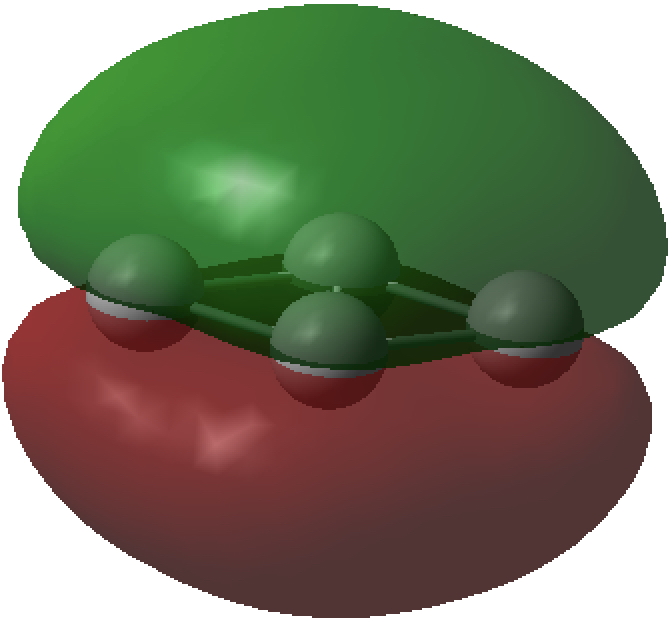## More tetrahedral fun. Spherical aromaticity (and other oddities) in N4 and C4 systems?

The thread thus far. The post about Na2He introduced the electride anionic counter-ion to Na+ as corresponding topologically to a rare feature known as a non-nuclear attractor. This prompted speculation about other systems with such a feature, and the focus shifted to a tetrahedral arrangement of four hydrogen atoms as a dication, sharing a total of two valence electrons. The story now continues here.

What emerged during comments about H42+ was that a density functional (DFT) derived wavefunction seemed to predict it to be a stable minimum, but that wavefunctions derived from coupled cluster or CASSCF methods predicted it to be a three-fold degenerate transition state instead. So I asked myself if perhaps other similar tetrahedral molecules less susceptible to such method ambiguity might be found. Here I record some of the species I investigated.

1. N4 in a tetrahedral allotropic arrangement of the element (ωB97XD/Def2-TZVPP DFT method: 10.14469/hpc/2217 and CCSD(T)/Def2-TZVPP 10.14469/hpc/2216). I found this intriguing, because each nitrogen has a lone pair of electrons and such an arrangement of eight electrons might be spherically aromatic according to the rule: 2(n+1)2, where n=1. Nitself is indeed a true minimum (rN-N  1.460Å) with all positive force constants at both the DFT (767, 1005 and 1443) and CCSD(T) (726, 940 and 1304 cm-1) levels, but with a free energy ~185 kcal/mol higher than dinitrogen. The electronic topology is uneventfully classical, with six line (bond) critical points along each N-N axis (magenta), four ring critical points (green) and one cage point (inner blue sphere); there is no non-nuclear attractor present.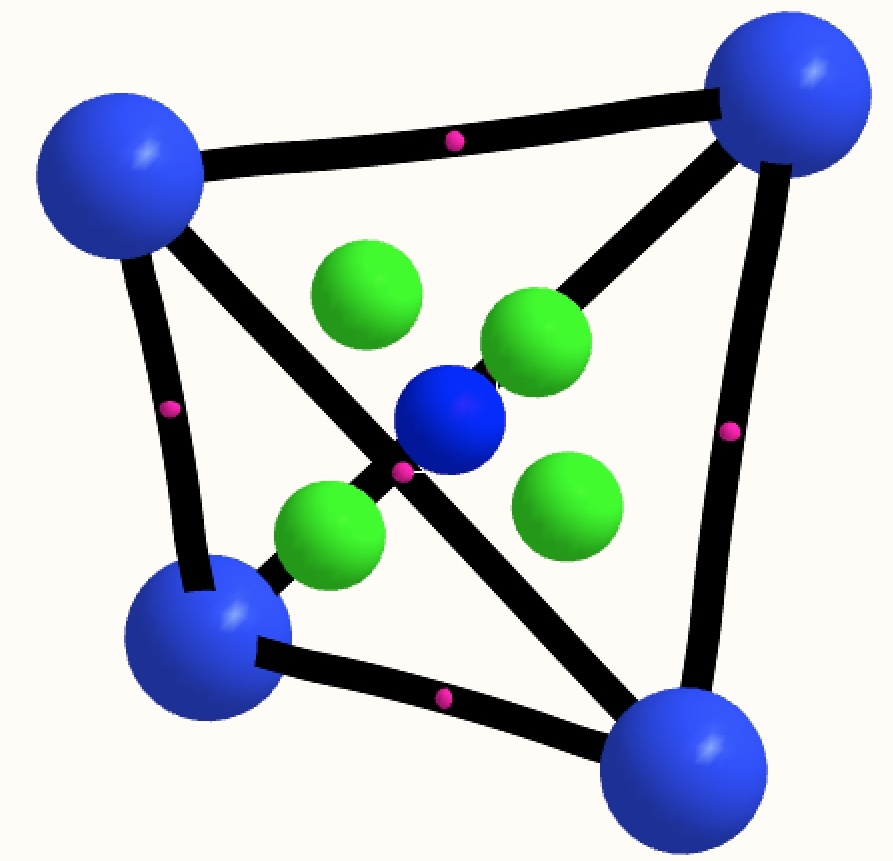The NICS value at the centre of the tetrahedron (coincident with the cage critical point) is -73 ppm, which does suggest aromaticity.
2. C4 in a tetrahedral allotropic arrangement of this element is also a minimum as closed shell singlet (rC-C 1.646Å) again with positive force constants (ωB97XD/Def2-TZVPP DFT, 10.14469/hpc/2224, 434, 715, 1052 cm-1) and the same electronic topology as N4.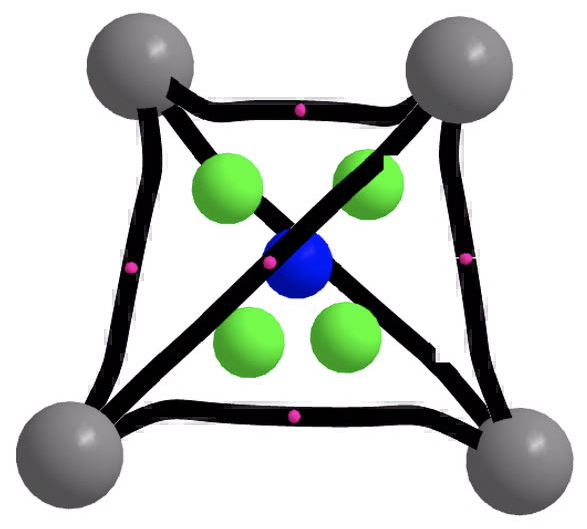The magnetic shielding at the ring centre is -1685 ppm, a value clearly perturbed by core ring currents or other factors; the molecule does not map to the 2(n+1)2 spherical aromaticity rule, which only allows values of 2,8,18, 32… electrons. I tried applying the ELF procedure using the computed WFN file (either direct or symmetrised, using both TopMod and MultiWFN) but the results did not have Td symmetry.
3. C42+ with two fewer electrons is also a minimum as a closed shell singlet (rC-C 1.521Å) tetrahedral species (ωB97XD/Def2-TZVPP: 10.14469/hpc/2218, 1132, 1136, 1448 cm-1; CCSD(T)/Def2-TZVPP 10.14469/hpc/2225 showing rather different normal mode energies of ~330, 592, 1126 cm-1 ) which can be thought as mapping to the spherical aromaticity formula 2(n+1)2, where n=0. The electronic topology is slightly different from C4 itself, with four ring points (green) very close to the cage point in the centre.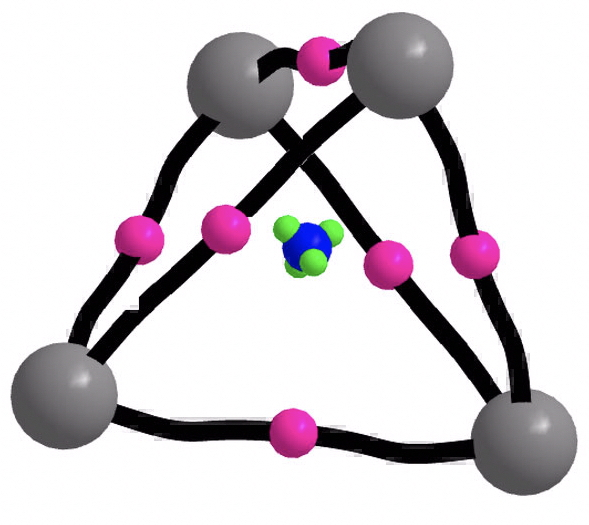The ELF function now behaves itself in terms of symmetry, and produces a result in fact very similar to the H42+ molecule which started this topic rolling. There is an ELF basin with 0.14e located in the centroid and six equivalent basins (2.25e) spanning each pair of carbon atoms, although these C-C bonds are hugely banana shaped! That central electron basin closely resembles the one found in H42+ itself. The magnetic shielding at the centre of 3349 ppm is not meaningful in deciding if the molecule is indeed “aromatic”.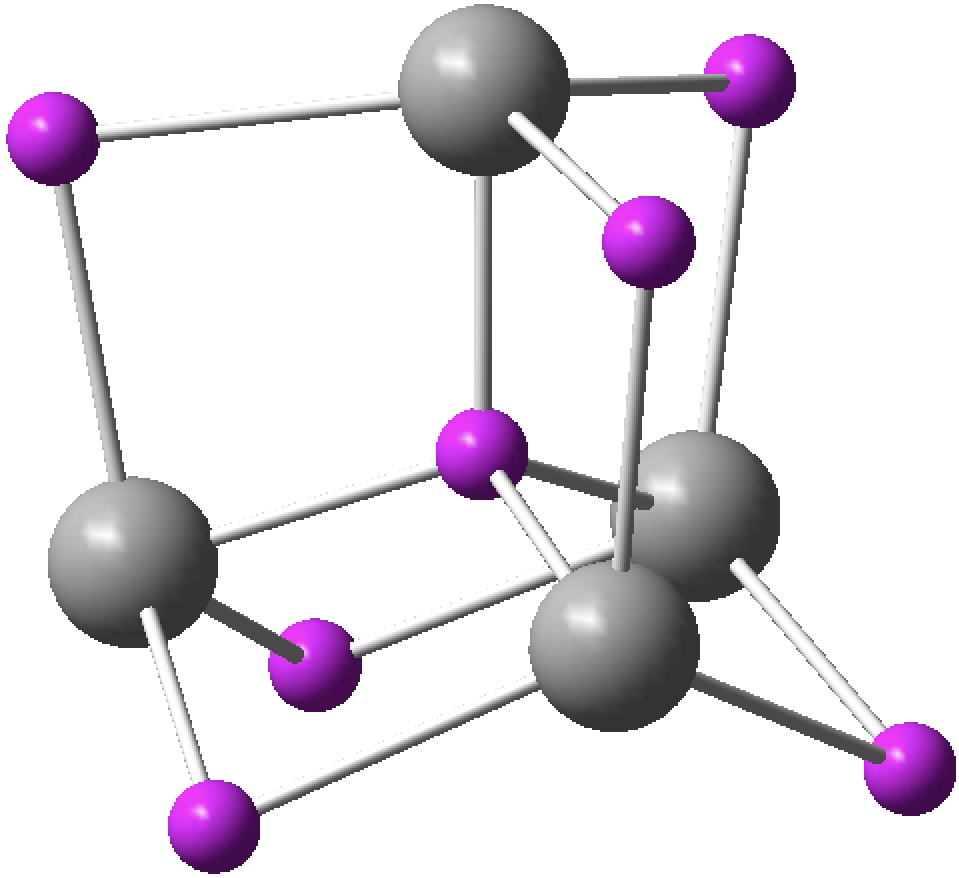4. C41-  is again a tetrahedral minimum, this time as a quartet 4A1 state (ωB97XD/Def2-TZVPP: 10.14469/hpc/2219, 918, 1024, 1377 cm-1; CCSD(T)/Def2-TZVPP 10.14469/hpc/2237, 824, 895, 1303 cm-1). The electronic topology is the same as before.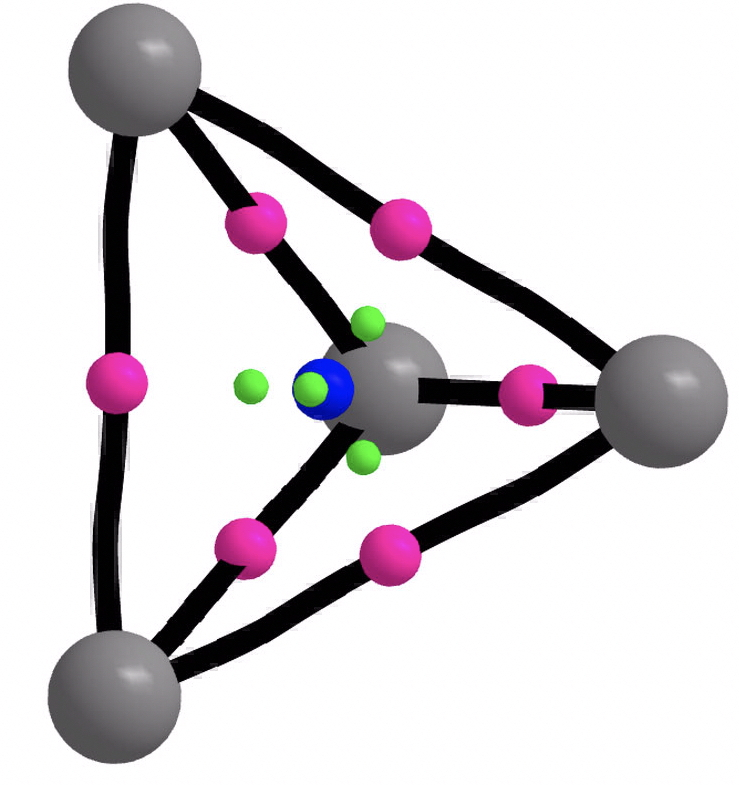Open shell spherical aromaticity is given by the 2N2 + 2N + 1 (with S = N + ½) rule. A quartet state has S=3/2, hence N=1 and the formula stipulates 5 delocalizable electrons for aromaticity, which this species has! The isotropic magnetic shielding is 695 ppm, which again is not immediately helpful.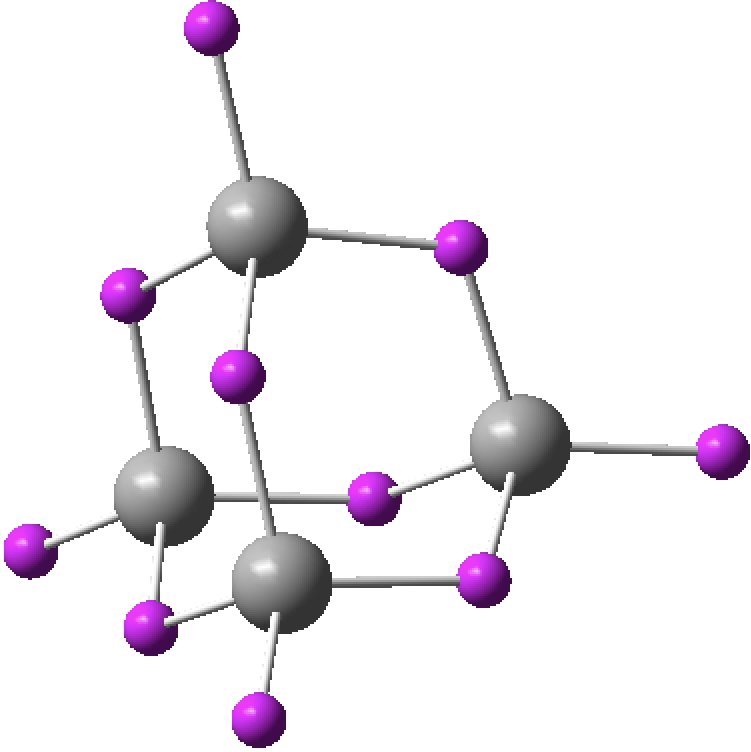The ELF analysis ((above) shows just two types of basin, with four “lone pairs” at each carbon vertex (1.24e) and eight associated with the C-C “bent” bonds (1.95e).

What did I learn?

• Firstly, that the (very unstable) tetrahedral allotrope of nitrogen might be a spherical aromatic.
• Secondly, that tetrahedral closed-shell singlet C4 has a very odd wavefunction; this needs further work.
• Thirdly that tetrahedral C42+  closely resembles H42+  in having a basin of electrons at the very centre, but that unlike H42+ it does appear to be a stable minimum.
• Finally, that the radical anion C4 might be perhaps the smallest possible example of an open shell spherical aromatic.

And perhaps also in trying to answer some simple questions, I have also raised several more puzzles. Onwards and occasionally upwards.

This wavefunction is clearly odd, and needs further analysis.

## References

1. A. Hirsch, Z. Chen, and H. Jiao, "Spherical Aromaticity inIh Symmetrical Fullerenes: The 2(N+1)2 Rule", Angewandte Chemie, vol. 39, pp. 3915-3917, 2000. http://dx.doi.org/10.1002/1521-3773(20001103)39:21<3915::AID-ANIE3915>3.0.CO;2-O
2. J. Poater, and M. Solà, "Open-shell spherical aromaticity: the 2N2 + 2N + 1 (with S = N + ½) rule", Chemical Communications, vol. 47, pp. 11647, 2011. http://dx.doi.org/10.1039/C1CC14958J

### One Response to “More tetrahedral fun. Spherical aromaticity (and other oddities) in N4 and C4 systems?”

1.Henry Rzepa says:

In my concluding remarks above, I noted that the wavefunction for tetrahedral C4 was problematic. Following this thread;

1. A CCSD(T)/Def2-TZVPP optimisation results in a tetrahedral geometry (rC-C 1.646Å) with all positive force constants (DOI: 10.14469/hpc/2226, ν ~85, 573, 1011 cm-1).
2. But the instability of the density as manifested in my ELF results suggests that the molecule may have strong multireference character. Hence next a CASSCF(8,9)/Def2-TZVPP calculation (DOI: 10.14469/hpc/2227) which reduces the symmetry to Cs as shown below. The singlet wavefunction is indeed multireference, with the three largest configurations each having contributions of 31.4% and 6% from others.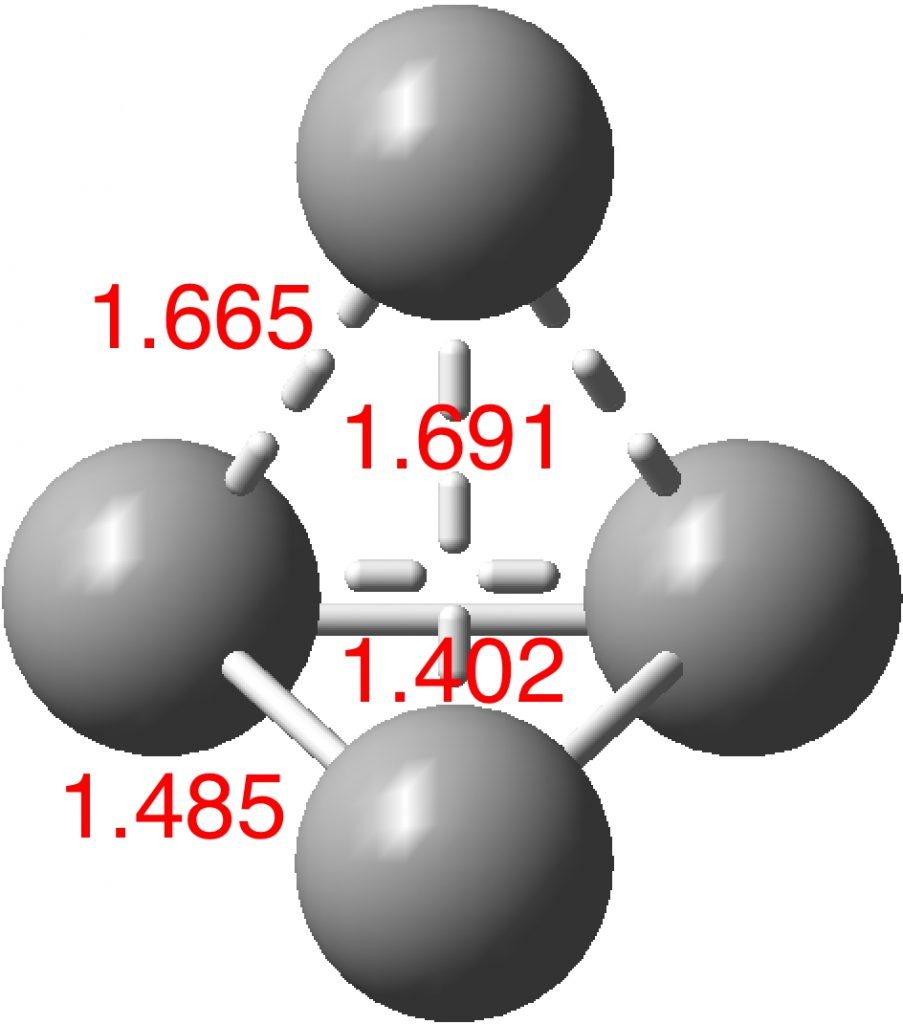3. If this geometry is then used for a conventional single reference DFT optimisation one gets the equally intriguing planar structure shown below, with the ELF analysis following.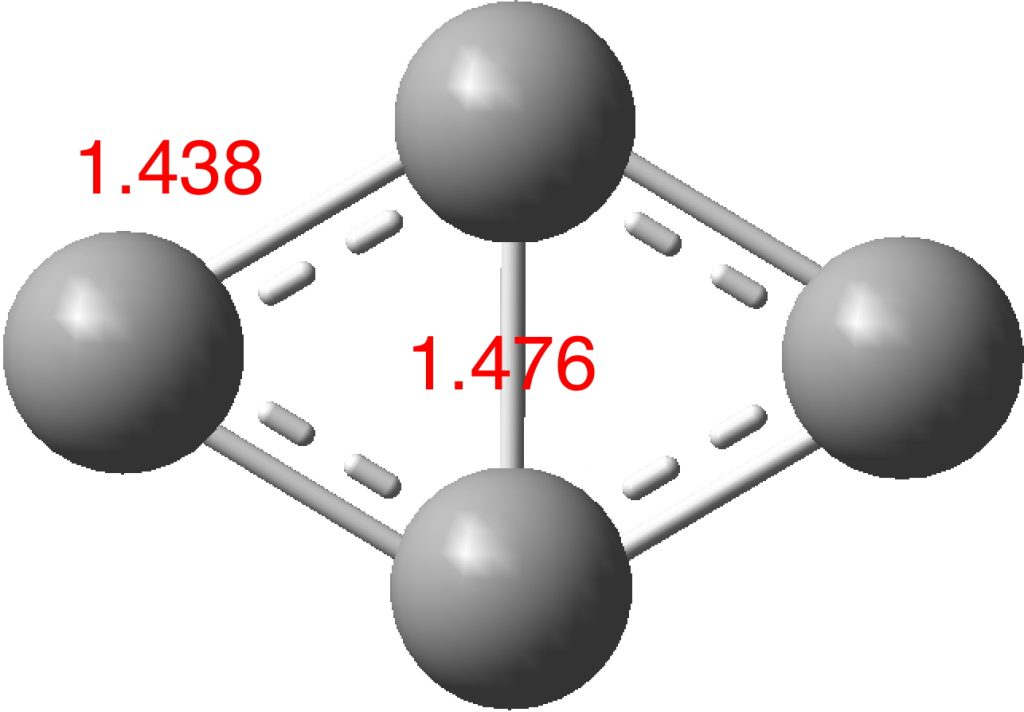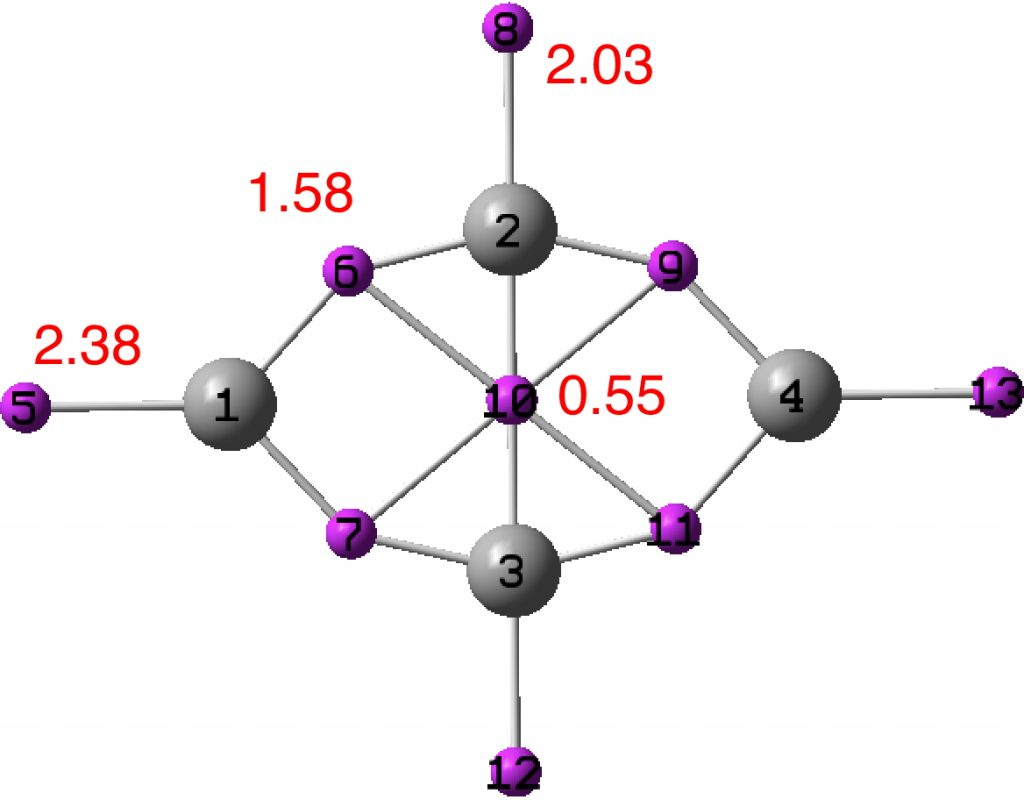The presence of just two π-electrons makes this species a two dimensional π-aromatic, but it is the σ-electrons that intrigue more in this example.